Publicité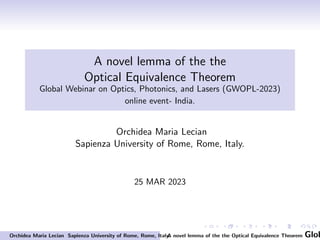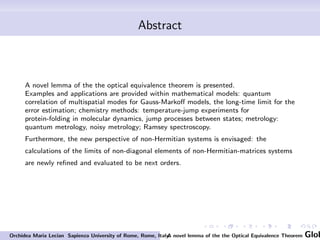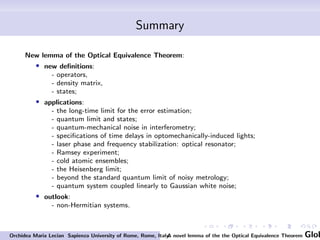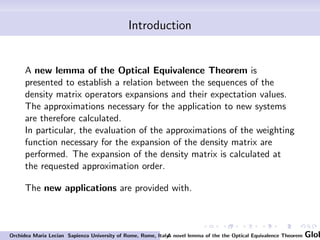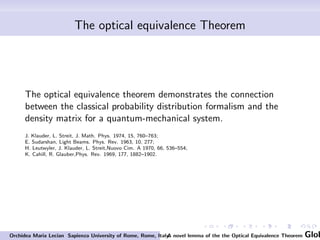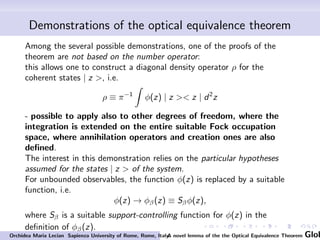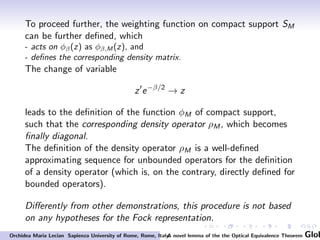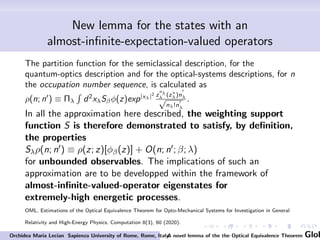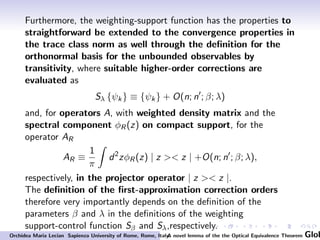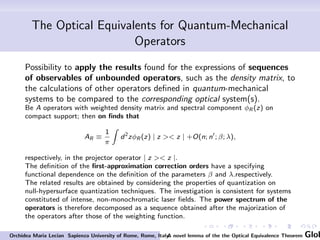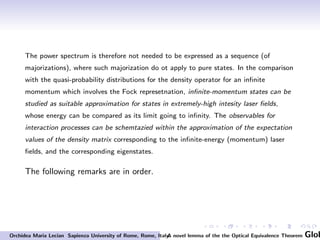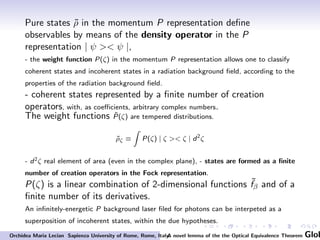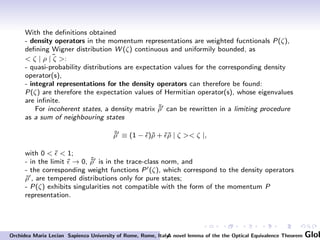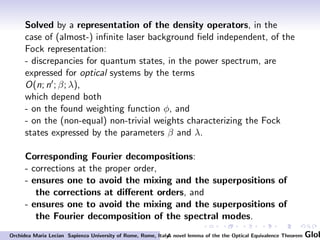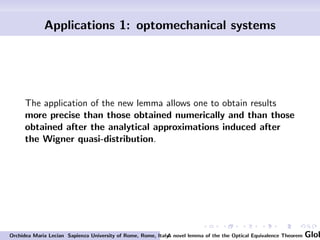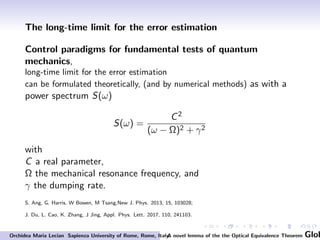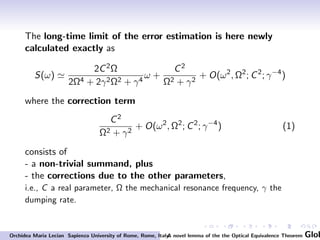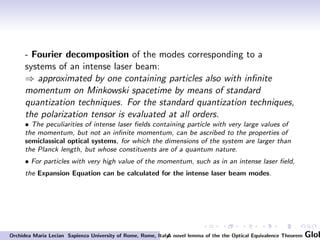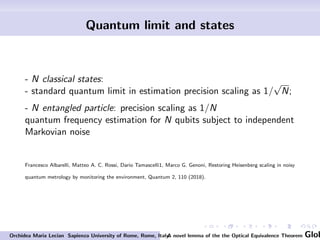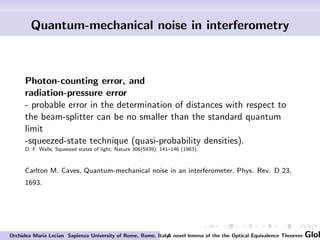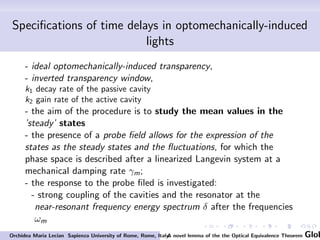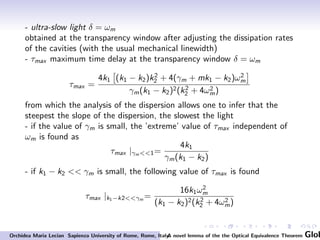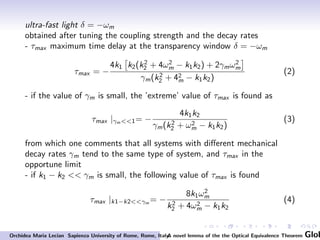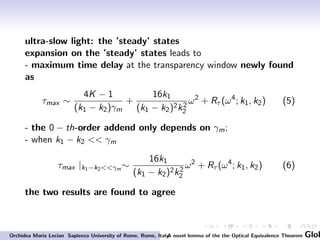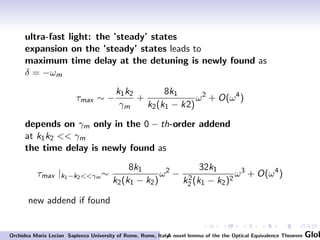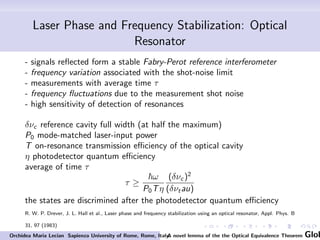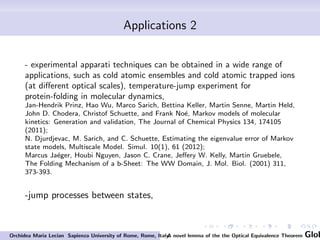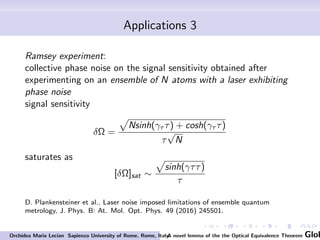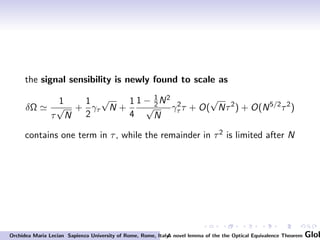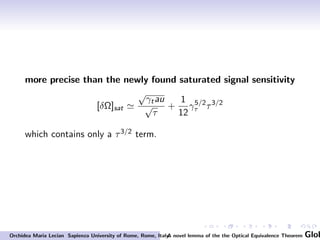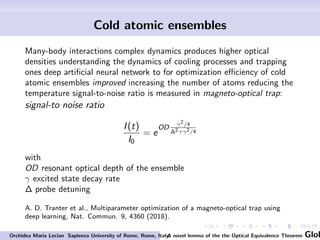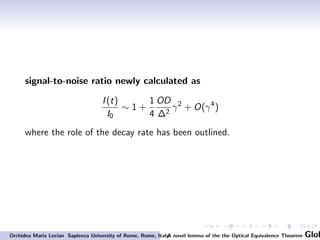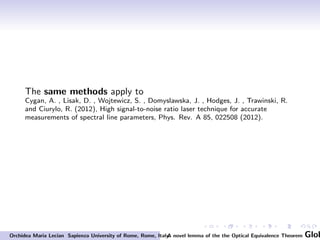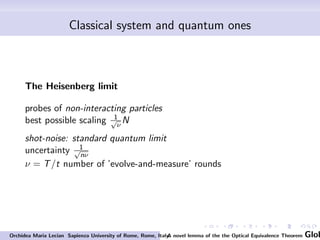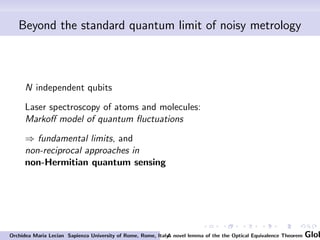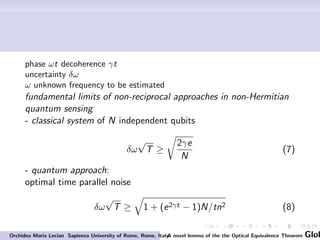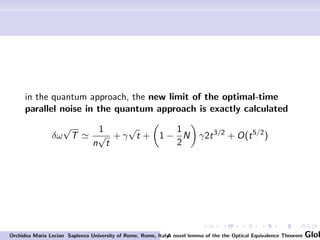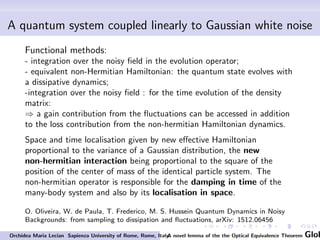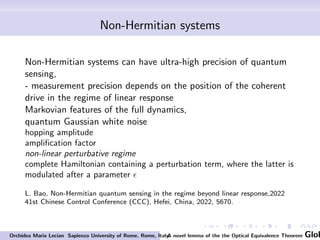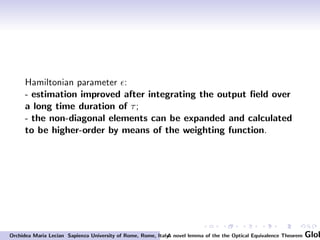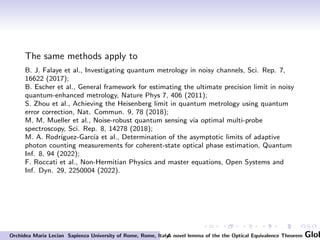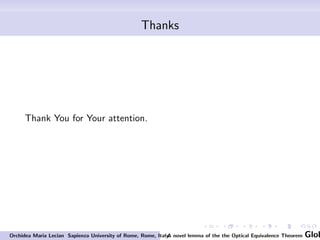1 sur 43
Publicité

### A novel lemma of the Optical Equivalence Theorem

1. A novel lemma of the the Optical Equivalence Theorem Global Webinar on Optics, Photonics, and Lasers (GWOPL-2023) online event- India. Orchidea Maria Lecian Sapienza University of Rome, Rome, Italy. 25 MAR 2023 Orchidea Maria Lecian Sapienza University of Rome, Rome, Italy. A novel lemma of the the Optical Equivalence Theorem Glob
2. Abstract A novel lemma of the the optical equivalence theorem is presented. Examples and applications are provided within mathematical models: quantum correlation of multispatial modes for Gauss-Markoff models, the long-time limit for the error estimation; chemistry methods: temperature-jump experiments for protein-folding in molecular dynamics, jump processes between states; metrology: quantum metrology, noisy metrology; Ramsey spectroscopy. Furthermore, the new perspective of non-Hermitian systems is envisaged: the calculations of the limits of non-diagonal elements of non-Hermitian-matrices systems are newly refined and evaluated to be next orders. Orchidea Maria Lecian Sapienza University of Rome, Rome, Italy. A novel lemma of the the Optical Equivalence Theorem Glob
3. Summary New lemma of the Optical Equivalence Theorem: • new definitions: - operators, - density matrix, - states; • applications: - the long-time limit for the error estimation; - quantum limit and states; - quantum-mechanical noise in interferometry; - specifications of time delays in optomechanically-induced lights; - laser phase and frequency stabilization: optical resonator; - Ramsey experiment; - cold atomic ensembles; - the Heisenberg limit; - beyond the standard quantum limit of noisy metrology; - quantum system coupled linearly to Gaussian white noise; • outlook: - non-Hermitian systems. Orchidea Maria Lecian Sapienza University of Rome, Rome, Italy. A novel lemma of the the Optical Equivalence Theorem Glob
4. Introduction A new lemma of the Optical Equivalence Theorem is presented to establish a relation between the sequences of the density matrix operators expansions and their expectation values. The approximations necessary for the application to new systems are therefore calculated. In particular, the evaluation of the approximations of the weighting function necessary for the expansion of the density matrix are performed. The expansion of the density matrix is calculated at the requested approximation order. The new applications are provided with. Orchidea Maria Lecian Sapienza University of Rome, Rome, Italy. A novel lemma of the the Optical Equivalence Theorem Glob
5. The optical equivalence Theorem The optical equivalence theorem demonstrates the connection between the classical probability distribution formalism and the density matrix for a quantum-mechanical system. J. Klauder, L. Streit, J. Math. Phys. 1974, 15, 760–763; E. Sudarshan, Light Beams. Phys. Rev. 1963, 10, 277; H. Leutwyler, J. Klauder, L. Streit,Nuovo Cim. A 1970, 66, 536–554; K. Cahill, R. Glauber,Phys. Rev. 1969, 177, 1882–1902. Orchidea Maria Lecian Sapienza University of Rome, Rome, Italy. A novel lemma of the the Optical Equivalence Theorem Glob
6. Demonstrations of the optical equivalence theorem Among the several possible demonstrations, one of the proofs of the theorem are not based on the number operator: this allows one to construct a diagonal density operator ρ for the coherent states | z >, i.e. ρ ≡ π−1 Z ϕ(z) | z >< z | d2 z - possible to apply also to other degrees of freedom, where the integration is extended on the entire suitable Fock occupation space, where annihilation operators and creation ones are also defined. The interest in this demonstration relies on the particular hypotheses assumed for the states | z > of the system. For unbounded observables, the function ϕ(z) is replaced by a suitable function, i.e. ϕ(z) → ϕβ(z) ≡ Sβϕ(z), where Sβ is a suitable support-controlling function for ϕ(z) in the definition of ϕβ(z). Orchidea Maria Lecian Sapienza University of Rome, Rome, Italy. A novel lemma of the the Optical Equivalence Theorem Glob
7. To proceed further, the weighting function on compact support SM can be further defined, which - acts on ϕβ(z) as ϕβ,M (z), and - defines the corresponding density matrix. The change of variable z′ e−β/2 → z leads to the definition of the function ϕM of compact support, such that the corresponding density operator ρM, which becomes finally diagonal. The definition of the density operator ρM is a well-defined approximating sequence for unbounded operators for the definition of a density operator (which is, on the contrary, directly defined for bounded operators). Differently from other demonstrations, this procedure is not based on any hypotheses for the Fock representation. Orchidea Maria Lecian Sapienza University of Rome, Rome, Italy. A novel lemma of the the Optical Equivalence Theorem Glob
8. New lemma for the states with an almost-infinite-expectation-valued operators The partition function for the semiclassical description, for the quantum-optics description and for the optical-systems descriptions, for n the occupation number sequence, is calculated as ρ(n; n′ ) ≡ Πλ R d2 xλSβϕ(z)exp|xλ|2 z nλ λ (z∗ λ)n ′ λ √ nλ!n ′ λ . In all the approximation here described, the weighting support function S is therefore demonstrated to satisfy, by definition, the properties Sλρ(n; n′) ≡ ρ(z; z)[ϕβ(z)] + O(n; n′; β; λ) for unbounded observables. The implications of such an approximation are to be developped within the framework of almost-infinite-valued-operator eigenstates for extremely-high energetic processes. OML, Estimations of the Optical Equivalence Theorem for Opto-Mechanical Systems for Investigation in General Relativity and High-Energy Physics, Computation 8(3), 60 (2020). Orchidea Maria Lecian Sapienza University of Rome, Rome, Italy. A novel lemma of the the Optical Equivalence Theorem Glob
9. Furthermore, the weighting-support function has the properties to straightforward be extended to the convergence properties in the trace class norm as well through the definition for the orthonormal basis for the unbounded observables by transitivity, where suitable higher-order corrections are evaluated as Sλ {ψk} ≡ {ψk} + O(n; n′ ; β; λ) and, for operators A, with weighted density matrix and the spectral component ϕR(z) on compact support, for the operator AR AR ≡ 1 π Z d2 zϕR(z) | z >< z | +O(n; n′ ; β; λ), respectively, in the projector operator | z >< z |. The definition of the first-approximation correction orders therefore very importantly depends on the definition of the parameters β and λ in the definitions of the weighting support-control function Sβ and Sλ,respectively. Orchidea Maria Lecian Sapienza University of Rome, Rome, Italy. A novel lemma of the the Optical Equivalence Theorem Glob
10. The Optical Equivalents for Quantum-Mechanical Operators Possibility to apply the results found for the expressions of sequences of observables of unbounded operators, such as the density matrix, to the calculations of other operators defined in quantum-mechanical systems to be compared to the corresponding optical system(s). Be A operators with weighted density matrix and spectral component ϕR (z) on compact support; then on finds that AR ≡ 1 π Z d2 zϕR (z) | z >< z | +O(n; n′ ; β; λ), respectively, in the projector operator | z >< z |. The definition of the first-approximation correction orders have a specifying functional dependence on the definition of the parameters β and λ.respectively. The related results are obtained by considering the properties of quantization on null-hypersurface quantization techniques. The investigation is consistent for systems constituted of intense, non-monochromatic laser fields. The power spectrum of the operators is therefore decomposed as a sequence obtained after the majorization of the operators after those of the weighting function. Orchidea Maria Lecian Sapienza University of Rome, Rome, Italy. A novel lemma of the the Optical Equivalence Theorem Glob
11. The power spectrum is therefore not needed to be expressed as a sequence (of majorizations), where such majorization do ot apply to pure states. In the comparison with the quasi-probability distributions for the density operator for an infinite momentum which involves the Fock represetnation, infinite-momentum states can be studied as suitable approximation for states in extremely-high intesity laser fields, whose energy can be compared as its limit going to infinity. The observables for interaction processes can be schemtazied within the approximation of the expectation values of the density matrix corresponding to the infinite-energy (momentum) laser fields, and the corresponding eigenstates. The following remarks are in order. Orchidea Maria Lecian Sapienza University of Rome, Rome, Italy. A novel lemma of the the Optical Equivalence Theorem Glob
12. Pure states ρ̃ in the momentum P representation define observables by means of the density operator in the P representation | ψ >< ψ |, - the weight function P(ζ) in the momentum P representation allows one to classify coherent states and incoherent states in a radiation background field, according to the properties of the radiation background field. - coherent states represented by a finite number of creation operators, with, as coefficients, arbitrary complex numbers. The weight functions P̃(ζ) are tempered distributions. ρ̃ζ ≡ Z P(ζ) | ζ >< ζ | d2 ζ - d2ζ real element of area (even in the complex plane), - states are formed as a finite number of creation operators in the Fock representation. P(ζ) is a linear combination of 2-dimensional functions ˜ fβ and of a finite number of its derivatives. An infinitely-energetic P background laser filed for photons can be interpeted as a superposition of incoherent states, within the due hypotheses. Orchidea Maria Lecian Sapienza University of Rome, Rome, Italy. A novel lemma of the the Optical Equivalence Theorem Glob
13. With the definitions obtained - density operators in the momentum representations are weighted fucntionals P(ζ), defining Wigner distribution W (ζ) continuous and uniformily bounded, as < ζ | ˜ ρ | ζ >: - quasi-probability distributions are expectation values for the corresponding density operator(s), - integral representations for the density operators can therefore be found: P(ζ) are therefore the expectation values of Hermitian operator(s), whose eigenvalues are infinite. For incoherent states, a density matrix ˜ ρ̃′ can be rewritten in a limiting procedure as a sum of neighbouring states ˜ ρ̃′ ≡ (1 − ϵ̃)ρ̃ + ϵ̃ρ̃ | ζ >< ζ |, with 0 < ϵ̃ < 1; - in the limit ϵ̃ → 0, ˜ ρ̃′ is in the trace-class norm, and - the corresponding weight functions P′(ζ), which correspond to the density operators ρ̃′, are tempered distributions only for pure states; - P(ζ) exhibits singularities not compatible with the form of the momentum P representation. Orchidea Maria Lecian Sapienza University of Rome, Rome, Italy. A novel lemma of the the Optical Equivalence Theorem Glob
14. Solved by a representation of the density operators, in the case of (almost-) infinite laser background field independent, of the Fock representation: - discrepancies for quantum states, in the power spectrum, are expressed for optical systems by the terms O(n; n′; β; λ), which depend both - on the found weighting function ϕ, and - on the (non-equal) non-trivial weights characterizing the Fock states expressed by the parameters β and λ. Corresponding Fourier decompositions: - corrections at the proper order, - ensures one to avoid the mixing and the superpositions of the corrections at different orders, and - ensures one to avoid the mixing and the superpositions of the Fourier decomposition of the spectral modes. Orchidea Maria Lecian Sapienza University of Rome, Rome, Italy. A novel lemma of the the Optical Equivalence Theorem Glob
15. Applications 1: optomechanical systems The application of the new lemma allows one to obtain results more precise than those obtained numerically and than those obtained after the analytical approximations induced after the Wigner quasi-distribution. Orchidea Maria Lecian Sapienza University of Rome, Rome, Italy. A novel lemma of the the Optical Equivalence Theorem Glob
16. The long-time limit for the error estimation Control paradigms for fundamental tests of quantum mechanics, long-time limit for the error estimation can be formulated theoretically, (and by numerical methods) as with a power spectrum S(ω) S(ω) = C2 (ω − Ω)2 + γ2 with C a real parameter, Ω the mechanical resonance frequency, and γ the dumping rate. S. Ang, G. Harris, W Bowen, M Tsang,New J. Phys. 2013, 15, 103028; J. Du, L. Cao, K. Zhang, J Jing, Appl. Phys. Lett. 2017, 110, 241103. Orchidea Maria Lecian Sapienza University of Rome, Rome, Italy. A novel lemma of the the Optical Equivalence Theorem Glob
17. The long-time limit of the error estimation is here newly calculated exactly as S(ω) ≃ 2C2Ω 2Ω4 + 2γ2Ω2 + γ4 ω + C2 Ω2 + γ2 + O(ω2 , Ω2 ; C2 ; γ−4 ) where the correction term C2 Ω2 + γ2 + O(ω2 , Ω2 ; C2 ; γ−4 ) (1) consists of - a non-trivial summand, plus - the corrections due to the other parameters, i.e., C a real parameter, Ω the mechanical resonance frequency, γ the dumping rate. Orchidea Maria Lecian Sapienza University of Rome, Rome, Italy. A novel lemma of the the Optical Equivalence Theorem Glob
18. - Fourier decomposition of the modes corresponding to a systems of an intense laser beam: ⇒ approximated by one containing particles also with infinite momentum on Minkowski spacetime by means of standard quantization techniques. For the standard quantization techniques, the polarization tensor is evaluated at all orders. • The peculiarities of intense laser fields containing particle with very large values of the momentum, but not an infinite momentum, can be ascribed to the properties of semiclassical optical systems, for which the dimensions of the system are larger than the Planck length, but whose constituents are of a quantum nature. • For particles with very high value of the momentum, such as in an intense laser field, the Expansion Equation can be calculated for the intense laser beam modes. Orchidea Maria Lecian Sapienza University of Rome, Rome, Italy. A novel lemma of the the Optical Equivalence Theorem Glob
19. Quantum limit and states - N classical states: - standard quantum limit in estimation precision scaling as 1/ √ N; - N entangled particle: precision scaling as 1/N quantum frequency estimation for N qubits subject to independent Markovian noise Francesco Albarelli, Matteo A. C. Rossi, Dario Tamascelli1, Marco G. Genoni, Restoring Heisenberg scaling in noisy quantum metrology by monitoring the environment, Quantum 2, 110 (2018). Orchidea Maria Lecian Sapienza University of Rome, Rome, Italy. A novel lemma of the the Optical Equivalence Theorem Glob
20. Quantum-mechanical noise in interferometry Photon-counting error, and radiation-pressure error - probable error in the determination of distances with respect to the beam-splitter can be no smaller than the standard quantum limit -squeezed-state technique (quasi-probability densities). D. F. Walls, Squeezed states of light, Nature 306(5939), 141–146 (1983). Carlton M. Caves, Quantum-mechanical noise in an interferometer, Phys. Rev. D 23, 1693. Orchidea Maria Lecian Sapienza University of Rome, Rome, Italy. A novel lemma of the the Optical Equivalence Theorem Glob
21. Specifications of time delays in optomechanically-induced lights - ideal optomechanically-induced transparency, - inverted transparency window, k1 decay rate of the passive cavity k2 gain rate of the active cavity - the aim of the procedure is to study the mean values in the ’steady’ states - the presence of a probe field allows for the expression of the states as the steady states and the fluctuations, for which the phase space is described after a linearized Langevin system at a mechanical damping rate γm; - the response to the probe filed is investigated: - strong coupling of the cavities and the resonator at the near-resonant frequency energy spectrum δ after the frequencies ωm Orchidea Maria Lecian Sapienza University of Rome, Rome, Italy. A novel lemma of the the Optical Equivalence Theorem Glob
22. - ultra-slow light δ = ωm obtained at the transparency window after adjusting the dissipation rates of the cavities (with the usual mechanical linewidth) - τmax maximum time delay at the transparency window δ = ωm τmax = 4k1 (k1 − k2)k2 2 + 4(γm + mk1 − k2)ω2 m γm(k1 − k2)2(k2 2 + 4ω2 m) from which the analysis of the dispersion allows one to infer that the steepest the slope of the dispersion, the slowest the light - if the value of γm is small, the ’extreme’ value of τmax independent of ωm is found as τmax |γm1= 4k1 γm(k1 − k2) - if k1 − k2 γm is small, the following value of τmax is found τmax |k1−k2γm = 16k1ω2 m (k1 − k2)2(k2 2 + 4ω2 m) Orchidea Maria Lecian Sapienza University of Rome, Rome, Italy. A novel lemma of the the Optical Equivalence Theorem Glob
23. ultra-fast light δ = −ωm obtained after tuning the coupling strength and the decay rates - τmax maximum time delay at the transparency window δ = −ωm τmax = − 4k1 k2(k2 2 + 4ω2 m − k1k2) + 2γmω2 m γm(k2 2 + 42 m − k1k2) (2) - if the value of γm is small, the ’extreme’ value of τmax is found as τmax |γm1= − 4k1k2 γm(k2 2 + ω2 m − k1k2) (3) from which one comments that all systems with different mechanical decay rates γm tend to the same type of system, and τmax in the opportune limit - if k1 − k2 γm is small, the following value of τmax is found τmax |k1−k2γm = − 8k1ω2 m k2 2 + 4ω2 m − k1k2 (4) Orchidea Maria Lecian Sapienza University of Rome, Rome, Italy. A novel lemma of the the Optical Equivalence Theorem Glob
24. ultra-slow light: the ’steady’ states expansion on the ’steady’ states leads to - maximum time delay at the transparency window newly found as τmax ∼ 4K − 1 (k1 − k2)γm + 16k1 (k1 − k2)2k2 2 ω2 + Rτ (ω4 ; k1, k2) (5) - the 0 − th-order addend only depends on γm; - when k1 − k2 γm τmax |k1−k2γm ∼ 16k1 (k1 − k2)2k2 2 ω2 + Rτ (ω4 ; k1, k2) (6) the two results are found to agree Orchidea Maria Lecian Sapienza University of Rome, Rome, Italy. A novel lemma of the the Optical Equivalence Theorem Glob
25. ultra-fast light: the ’steady’ states expansion on the ’steady’ states leads to maximum time delay at the detuning is newly found as δ = −ωm τmax ∼ − k1k2 γm + 8k1 k2(k1 − k2) ω2 + O(ω4 ) depends on γm only in the 0 − th-order addend at k1k2 γm the time delay is newly found as τmax |k1−k2γm ∼ 8k1 k2(k1 − k2) ω2 − 32k1 k2 2 (k1 − k2)2 ω3 + O(ω4 ) new addend if found Orchidea Maria Lecian Sapienza University of Rome, Rome, Italy. A novel lemma of the the Optical Equivalence Theorem Glob
26. Laser Phase and Frequency Stabilization: Optical Resonator - signals reflected form a stable Fabry-Perot reference interferometer - frequency variation associated with the shot-noise limit - measurements with average time τ - frequency fluctuations due to the measurement shot noise - high sensitivity of detection of resonances δνc reference cavity full width (at half the maximum) P0 mode-matched laser-input power T on-resonance transmission efficiency of the optical cavity η photodetector quantum efficiency average of time τ τ ≥ ℏω P0Tη (δνc )2 (δνtau) the states are discrimined after the photodetector quantum efficiency R. W. P. Drever, J. L. Hall et al., Laser phase and frequency stabilization using an optical resonator, Appl. Phys. B 31, 97 (1983) Orchidea Maria Lecian Sapienza University of Rome, Rome, Italy. A novel lemma of the the Optical Equivalence Theorem Glob
27. Applications 2 - experimental apparati techniques can be obtained in a wide range of applications, such as cold atomic ensembles and cold atomic trapped ions (at different optical scales), temperature-jump experiment for protein-folding in molecular dynamics, Jan-Hendrik Prinz, Hao Wu, Marco Sarich, Bettina Keller, Martin Senne, Martin Held, John D. Chodera, Christof Schuette, and Frank Noé, Markov models of molecular kinetics: Generation and validation, The Journal of Chemical Physics 134, 174105 (2011); N. Djurdjevac, M. Sarich, and C. Schuette, Estimating the eigenvalue error of Markov state models, Multiscale Model. Simul. 10(1), 61 (2012); Marcus Jaéger, Houbi Nguyen, Jason C. Crane, Jeffery W. Kelly, Martin Gruebele, The Folding Mechanism of a b-Sheet: The WW Domain, J. Mol. Biol. (2001) 311, 373-393. -jump processes between states, Orchidea Maria Lecian Sapienza University of Rome, Rome, Italy. A novel lemma of the the Optical Equivalence Theorem Glob
28. - quantum correlation of multispatial modes for Gauss-Markoff models, Du, J.; Cao, L.; Zhang, K.; Jing, J. Experimental observation of multi-spatial-mode quantum correlations in four-wave mixing with a conical pump and a conical probe. Appl. Phys. Lett. 2017, 110, 241103. - uncertainties estimations in quantum metrology, in decoherence and dissipation and for mixed system-collections for new highly-intense laser fields after the application for the of the pertinent expansions after the calculations. i.e. to be appplied to noisy metrology beyond the standard quantum limit. R. Chaves, J. B. Brask, M. Markiewicz, J. Kolodynski, A. Acin, Noisy metrology beyond the standard quantum limits, Phys. Rev. Lett. 111, 120401 (2013) [arxiv:1212.3286]; M. Napolitano, M.W. Mitchell, Nonlinear metrology with a quantum interface, New Journ. of Phys. 12, (2010). Orchidea Maria Lecian Sapienza University of Rome, Rome, Italy. A novel lemma of the the Optical Equivalence Theorem Glob
29. Applications 3 Ramsey experiment: collective phase noise on the signal sensitivity obtained after experimenting on an ensemble of N atoms with a laser exhibiting phase noise signal sensitivity δΩ = p Nsinh(γτ τ) + cosh(γτ τ) τ √ N saturates as [δΩ]sat ∼ p sinh(γττ) τ D. Plankensteiner et al., Laser noise imposed limitations of ensemble quantum metrology, J. Phys. B: At. Mol. Opt. Phys. 49 (2016) 245501. Orchidea Maria Lecian Sapienza University of Rome, Rome, Italy. A novel lemma of the the Optical Equivalence Theorem Glob
30. the signal sensibility is newly found to scale as δΩ ≃ 1 τ √ N + 1 2 γτ √ N + 1 4 1 − 1 2N2 √ N γ2 τ τ + O( √ Nτ2 ) + O(N5/2 τ2 ) contains one term in τ, while the remainder in τ2 is limited after N Orchidea Maria Lecian Sapienza University of Rome, Rome, Italy. A novel lemma of the the Optical Equivalence Theorem Glob
31. more precise than the newly found saturated signal sensitivity [δΩ]sat ≃ √ γtau √ τ + 1 12 γ5/2 τ τ3/2 which contains only a τ3/2 term. Orchidea Maria Lecian Sapienza University of Rome, Rome, Italy. A novel lemma of the the Optical Equivalence Theorem Glob
32. Cold atomic ensembles Many-body interactions complex dynamics produces higher optical densities understanding the dynamics of cooling processes and trapping ones deep artificial neural network to for optimization efficiency of cold atomic ensembles improved increasing the number of atoms reducing the temperature signal-to-noise ratio is measured in magneto-optical trap: signal-to noise ratio I(t) I0 = e OD γ2/4 ∆2+γ2/4 with OD resonant optical depth of the ensemble γ excited state decay rate ∆ probe detuning A. D. Tranter et al., Multiparameter optimization of a magneto-optical trap using deep learning, Nat. Commun. 9, 4360 (2018). Orchidea Maria Lecian Sapienza University of Rome, Rome, Italy. A novel lemma of the the Optical Equivalence Theorem Glob
33. signal-to-noise ratio newly calculated as I(t) I0 ∼ 1 + 1 4 OD ∆2 γ2 + O(γ4 ) where the role of the decay rate has been outlined. Orchidea Maria Lecian Sapienza University of Rome, Rome, Italy. A novel lemma of the the Optical Equivalence Theorem Glob
34. The same methods apply to Cygan, A. , Lisak, D. , Wojtewicz, S. , Domyslawska, J. , Hodges, J. , Trawinski, R. and Ciurylo, R. (2012), High signal-to-noise ratio laser technique for accurate measurements of spectral line parameters, Phys. Rev. A 85, 022508 (2012). Orchidea Maria Lecian Sapienza University of Rome, Rome, Italy. A novel lemma of the the Optical Equivalence Theorem Glob
35. Classical system and quantum ones The Heisenberg limit probes of non-interacting particles best possible scaling 1 √ ν N shot-noise: standard quantum limit uncertainty 1 √ nν ν = T/t number of ’evolve-and-measure’ rounds Orchidea Maria Lecian Sapienza University of Rome, Rome, Italy. A novel lemma of the the Optical Equivalence Theorem Glob
36. Beyond the standard quantum limit of noisy metrology N independent qubits Laser spectroscopy of atoms and molecules: Markoff model of quantum fluctuations ⇒ fundamental limits, and non-reciprocal approaches in non-Hermitian quantum sensing Orchidea Maria Lecian Sapienza University of Rome, Rome, Italy. A novel lemma of the the Optical Equivalence Theorem Glob
37. phase ωt decoherence γt uncertainty δω ω unknown frequency to be estimated fundamental limits of non-reciprocal approaches in non-Hermitian quantum sensing - classical system of N independent qubits δω √ T ≥ r 2γe N (7) - quantum approach: optimal time parallel noise δω √ T ≥ q 1 + (e2γt − 1)N/tn2 (8) Orchidea Maria Lecian Sapienza University of Rome, Rome, Italy. A novel lemma of the the Optical Equivalence Theorem Glob
38. in the quantum approach, the new limit of the optimal-time parallel noise in the quantum approach is exactly calculated δω √ T ≃ 1 n √ t + γ √ t + 1 − 1 2 N γ2t3/2 + O(t5/2 ) Orchidea Maria Lecian Sapienza University of Rome, Rome, Italy. A novel lemma of the the Optical Equivalence Theorem Glob
39. A quantum system coupled linearly to Gaussian white noise Functional methods: - integration over the noisy field in the evolution operator; - equivalent non-Hermitian Hamiltonian: the quantum state evolves with a dissipative dynamics; -integration over the noisy field : for the time evolution of the density matrix: ⇒ a gain contribution from the fluctuations can be accessed in addition to the loss contribution from the non-hermitian Hamiltonian dynamics. Space and time localisation given by new effective Hamiltonian proportional to the variance of a Gaussian distribution, the new non-hermitian interaction being proportional to the square of the position of the center of mass of the identical particle system. The non-hermitian operator is responsible for the damping in time of the many-body system and also by its localisation in space. O. Oliveira, W. de Paula, T. Frederico, M. S. Hussein Quantum Dynamics in Noisy Backgrounds: from sampling to dissipation and fluctuations, arXiv: 1512.06456 Orchidea Maria Lecian Sapienza University of Rome, Rome, Italy. A novel lemma of the the Optical Equivalence Theorem Glob
40. Non-Hermitian systems Non-Hermitian systems can have ultra-high precision of quantum sensing, - measurement precision depends on the position of the coherent drive in the regime of linear response Markovian features of the full dynamics, quantum Gaussian white noise hopping amplitude amplification factor non-linear perturbative regime complete Hamiltonian containing a perturbation term, where the latter is modulated after a parameter ϵ L. Bao, Non-Hermitian quantum sensing in the regime beyond linear response,2022 41st Chinese Control Conference (CCC), Hefei, China, 2022, 5670. Orchidea Maria Lecian Sapienza University of Rome, Rome, Italy. A novel lemma of the the Optical Equivalence Theorem Glob
41. Hamiltonian parameter ϵ: - estimation improved after integrating the output field over a long time duration of τ; - the non-diagonal elements can be expanded and calculated to be higher-order by means of the weighting function. Orchidea Maria Lecian Sapienza University of Rome, Rome, Italy. A novel lemma of the the Optical Equivalence Theorem Glob
42. The same methods apply to B. J. Falaye et al., Investigating quantum metrology in noisy channels, Sci. Rep. 7, 16622 (2017); B. Escher et al., General framework for estimating the ultimate precision limit in noisy quantum-enhanced metrology, Nature Phys 7, 406 (2011); S. Zhou et al., Achieving the Heisenberg limit in quantum metrology using quantum error correction, Nat. Commun. 9, 78 (2018); M. M. Mueller et al., Noise-robust quantum sensing via optimal multi-probe spectroscopy, Sci. Rep. 8, 14278 (2018); M. A. Rodrı́guez-Garcı́a et al., Determination of the asymptotic limits of adaptive photon counting measurements for coherent-state optical phase estimation, Quantum Inf. 8, 94 (2022); F. Roccati et al., Non-Hermitian Physics and master equations, Open Systems and Inf. Dyn. 29, 2250004 (2022). Orchidea Maria Lecian Sapienza University of Rome, Rome, Italy. A novel lemma of the the Optical Equivalence Theorem Glob
43. Thanks Thank You for Your attention. Orchidea Maria Lecian Sapienza University of Rome, Rome, Italy. A novel lemma of the the Optical Equivalence Theorem Glob
Publicité